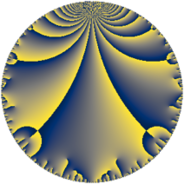# Properties

 Label 75.6.aLevel $75$ Weight $6$ Character orbit 75.a Rep. character $\chi_{75}(1,\cdot)$ Character field $\Q$ Dimension $15$ Newform subspaces $10$ Sturm bound $60$ Trace bound $2$

# Related objects

## Defining parameters

 Level: $$N$$ $$=$$ $$75 = 3 \cdot 5^{2}$$ Weight: $$k$$ $$=$$ $$6$$ Character orbit: $$[\chi]$$ $$=$$ 75.a (trivial) Character field: $$\Q$$ Newform subspaces: $$10$$ Sturm bound: $$60$$ Trace bound: $$2$$ Distinguishing $$T_p$$: $$2$$

## Dimensions

The following table gives the dimensions of various subspaces of $$M_{6}(\Gamma_0(75))$$.

Total New Old
Modular forms 56 15 41
Cusp forms 44 15 29
Eisenstein series 12 0 12

The following table gives the dimensions of the cuspidal new subspaces with specified eigenvalues for the Atkin-Lehner operators and the Fricke involution.

$$3$$$$5$$FrickeDim.
$$+$$$$+$$$$+$$$$3$$
$$+$$$$-$$$$-$$$$4$$
$$-$$$$+$$$$-$$$$5$$
$$-$$$$-$$$$+$$$$3$$
Plus space$$+$$$$6$$
Minus space$$-$$$$9$$

## Trace form

 $$15 q + 2 q^{2} + 9 q^{3} + 176 q^{4} + 54 q^{6} + 272 q^{7} + 60 q^{8} + 1215 q^{9} + O(q^{10})$$ $$15 q + 2 q^{2} + 9 q^{3} + 176 q^{4} + 54 q^{6} + 272 q^{7} + 60 q^{8} + 1215 q^{9} - 288 q^{11} + 828 q^{12} + 146 q^{13} + 2268 q^{14} + 3124 q^{16} - 3538 q^{17} + 162 q^{18} + 4442 q^{19} - 2214 q^{21} + 3444 q^{22} + 576 q^{23} - 1404 q^{24} + 20880 q^{26} + 729 q^{27} + 884 q^{28} - 11526 q^{29} - 15486 q^{31} + 25612 q^{32} + 10548 q^{33} - 23908 q^{34} + 14256 q^{36} - 12598 q^{37} - 59440 q^{38} + 1944 q^{39} - 23286 q^{41} - 29484 q^{42} + 38876 q^{43} + 48720 q^{44} + 3904 q^{46} + 19832 q^{47} + 59904 q^{48} + 71693 q^{49} - 33750 q^{51} - 63688 q^{52} - 53494 q^{53} + 4374 q^{54} + 16920 q^{56} - 14220 q^{57} + 71280 q^{58} - 33432 q^{59} - 87072 q^{61} + 46104 q^{62} + 22032 q^{63} - 172340 q^{64} - 124200 q^{66} + 54932 q^{67} - 244256 q^{68} - 15012 q^{69} + 210120 q^{71} + 4860 q^{72} - 20674 q^{73} - 282372 q^{74} + 23728 q^{76} - 52416 q^{77} + 133668 q^{78} - 32320 q^{79} + 98415 q^{81} - 3996 q^{82} - 103404 q^{83} + 55512 q^{84} - 203148 q^{86} + 14670 q^{87} + 335220 q^{88} + 234762 q^{89} + 29834 q^{91} + 172992 q^{92} + 101088 q^{93} + 640304 q^{94} + 107676 q^{96} - 134218 q^{97} - 327326 q^{98} - 23328 q^{99} + O(q^{100})$$

## Decomposition of $$S_{6}^{\mathrm{new}}(\Gamma_0(75))$$ into newform subspaces

Label Dim $A$ Field CM Traces A-L signs $q$-expansion
$a_{2}$ $a_{3}$ $a_{5}$ $a_{7}$ 3 5
75.6.a.a $1$ $12.029$ $$\Q$$ None $$-7$$ $$-9$$ $$0$$ $$-12$$ $+$ $+$ $$q-7q^{2}-9q^{3}+17q^{4}+63q^{6}-12q^{7}+\cdots$$
75.6.a.b $1$ $12.029$ $$\Q$$ None $$-4$$ $$-9$$ $$0$$ $$225$$ $+$ $+$ $$q-4q^{2}-9q^{3}-2^{4}q^{4}+6^{2}q^{6}+15^{2}q^{7}+\cdots$$
75.6.a.c $1$ $12.029$ $$\Q$$ None $$2$$ $$9$$ $$0$$ $$132$$ $-$ $+$ $$q+2q^{2}+9q^{3}-28q^{4}+18q^{6}+132q^{7}+\cdots$$
75.6.a.d $1$ $12.029$ $$\Q$$ None $$4$$ $$9$$ $$0$$ $$-225$$ $-$ $-$ $$q+4q^{2}+9q^{3}-2^{4}q^{4}+6^{2}q^{6}-15^{2}q^{7}+\cdots$$
75.6.a.e $1$ $12.029$ $$\Q$$ None $$6$$ $$-9$$ $$0$$ $$40$$ $+$ $+$ $$q+6q^{2}-9q^{3}+4q^{4}-54q^{6}+40q^{7}+\cdots$$
75.6.a.f $2$ $12.029$ $$\Q(\sqrt{89})$$ None $$-9$$ $$18$$ $$0$$ $$-108$$ $-$ $-$ $$q+(-4-\beta )q^{2}+9q^{3}+(6+9\beta )q^{4}+\cdots$$
75.6.a.g $2$ $12.029$ $$\Q(\sqrt{31})$$ None $$-6$$ $$-18$$ $$0$$ $$-102$$ $+$ $-$ $$q+(-3+\beta )q^{2}-9q^{3}+(8-6\beta )q^{4}+\cdots$$
75.6.a.h $2$ $12.029$ $$\Q(\sqrt{409})$$ None $$1$$ $$18$$ $$0$$ $$112$$ $-$ $+$ $$q+\beta q^{2}+9q^{3}+(70+\beta )q^{4}+9\beta q^{6}+\cdots$$
75.6.a.i $2$ $12.029$ $$\Q(\sqrt{31})$$ None $$6$$ $$18$$ $$0$$ $$102$$ $-$ $+$ $$q+(3+\beta )q^{2}+9q^{3}+(8+6\beta )q^{4}+(3^{3}+\cdots)q^{6}+\cdots$$
75.6.a.j $2$ $12.029$ $$\Q(\sqrt{89})$$ None $$9$$ $$-18$$ $$0$$ $$108$$ $+$ $-$ $$q+(5-\beta )q^{2}-9q^{3}+(15-9\beta )q^{4}+(-45+\cdots)q^{6}+\cdots$$

## Decomposition of $$S_{6}^{\mathrm{old}}(\Gamma_0(75))$$ into lower level spaces

$$S_{6}^{\mathrm{old}}(\Gamma_0(75)) \cong$$ $$S_{6}^{\mathrm{new}}(\Gamma_0(3))$$$$^{\oplus 3}$$$$\oplus$$$$S_{6}^{\mathrm{new}}(\Gamma_0(5))$$$$^{\oplus 4}$$$$\oplus$$$$S_{6}^{\mathrm{new}}(\Gamma_0(15))$$$$^{\oplus 2}$$$$\oplus$$$$S_{6}^{\mathrm{new}}(\Gamma_0(25))$$$$^{\oplus 2}$$